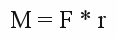# Torque

## Force

Force is a vector quantity pointing in a particular direction. Force is the cause for acceleration or deformation of bodies.
Omnipresent at the earth's surface is the force of gravitation. It is attracting all mass afflicted objects, accelerating them to the geocenter. Not later than at the earth's surface, the acceleration stops, because the normal force starts operating here. The normal force is caused by the deformation of the earth's surface. Like a compressed spring, the deformed surface effects compressive force pointing contrary to the gravitational force. If an object rests on a surface, gravitational and normal force have the same amount, but point at opposite directions. Let's have a look at a non point shaped body. The gravitational force (strictly spoken the sum of all gravitational forces) is pulling at the center of mass, the normal force at the point of contact. If both forces don't operate along the connection line of both points, the result is a

## Torque

Torque is a couple of parallel forces operating in contrary direction and therewith rotating or twisting a body.

Figure 1:
If a body is fixed at a revolvable axis, the resulting torque is:

[2.14]Where is:
M - Resulting torque,
r - distance between revolvable axis and operating force (lever),
F - force perpendicular to lever

Figure 2:
The left stone at the picture is falling free. The gravitational force and it's counterpart, the reaction force are operating at the center of mass. The sum of both forces is zero hence there is no torque.
If the stone is resting on a surface, the normal force prevents the stone from being accelerated by gravitational force. The normal force is operating at the point of contact pointing upwards. The gravitational force is still acting downwards from the center of mass. If the center of mass is exactly above the point of contact, there is no resulting torque and therefore no movement. This academic situation ends as soon as there is just a veeeeery little variance, leading to a resulting torque which starts tilting the stone. You won't find a stone resting on it's tip. At the right you can see the tilted stone resting on two contact points. As a result, normal forces are operating upwards at both points. The gravitational force is still pointing downwards. The sum of the normal forces (FN1 and FN2) equals the absolute value of the gravitational force (FG) and the sum of torques is zero. The stone is resting in a stable position. Painted two-dimensionally, the stone is a three-dimensional body, hence there will be a third contact point in reality. Even for the three points of contact the sum of gravitational force and normal forces leads to zero torque.
Figure 3:

## Lever

A rod-shaped, rigid body being fixed at a fulcrum is called a lever.
At the picture to the left, the lever is attached at point A. If the lever rests, the sum of all operating forces is zero:
F1 * s1 + F2 * s2 = 0 or F2 = - s1 / s2 * F1
if e.g. s1 is three times as long as s2 there is:
F2 = -(3 * s2) / s2 * F1 or F2 = -3 * F1
By using a lever, you can transform weak forces into strong forces. The disadvantage is, that you have to apply those lower forces over a longer distance, if you like to move an object. A lever is used to transform forces.
Note the forces operating at the fulcrum. Because of the fact that the lever doesn't move, the sum of all forces must be zero:
F1 + F2 + FA = 0 or FA = -(F1 + F2)
For the mentioned example there is:
FA = -(F1 + (-3 * F1)) = 2 * F1

Figure 4:

## Gear drive

A gear drive consists of at least two toothed wheels as you can see at the left picture. The left wheel consists of 12 and the right one 24 teeth. The diameter of the right wheel is twice as much as those of the left wheel. If the left wheel starts spinning with a torque of F * s1, the force F operates with the lever s2 at the right wheel. Therewith the torque of the right wheel is -F * s2. The sing (-) clarifies that the wheel is spinning contrary to the left wheel. Because of the fact that s2 is twice s1, the resulting torque is two times larger than those of the left wheel. The rotational speed is just half as big as those of the left wheel. Therewith the same amount of work is done by both wheels. The gear drive also works in the other way. If the right wheel is actuated, the left one rotates with double speed and just half the torque of the right one. A gear drive converts torque.
Figure 5:

## Wheel drive

While talking about torque, we considered the force operating at the (resting) fulcrum just as a side effect. While moving with wheel powered vehicles, the force operating at this bearing is pushing us forward.
At the left picture you can see the effects of torque caused by an engine and operating on a wheel. According to the torque, the force FM is operating at the contact surface of the tire. While FM is taller than the maximum amount of the static friction, the contact point marks the center of rotation and the wheel axle is pushed into the opposite direction by FL. The force operating at the axle is pushing the car.